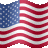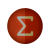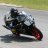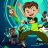# Confidence Mean Indicator#### J007RMC

##### Active member
2019 Donor
VIP
Calculates standard error and constructs Confidence Intervals for mean
Mobius
V01.01.01.2018
{Simple Confidence system. Trade is taken when close crosses the mean and added to when price is outside the confidence bands and trade is exited once close crosses into the bands.} Really know more of the indicator except that one can changed=number of days. The red labels indicate the Laguerre indicator a part of the Confidence Mean Indicator. and definitely dont understand the thumbs down lol.

Code:
``````# Confidence Intervals
# calculates standard error and constructs Confidence Intervals for mean
# Mobius
# V01.01.01.2018
# Simple Confidence system. Trade is taken when close crosses the mean and added to when price is outside the confidence bands and trade is exited once close crosses into the bands.

input n = 21;

# Detrended Mean
plot detrended_Mean = Average(close + (close - close[n/2]), n);
# variance of the mean
def var_of_mean = Sum(sqr(close - detrended_mean), n) / (n-1);
# standard deviation
def st_dev = sqrt(var_of_mean);
# standard error of the mean
def st_error = st_dev / sqrt(n);
# Determine the 95% Confidence Level
# Margin Of Error t-statistic of 1.96
def mar_of_error = 1.96 * st_error;
# Confidence Interval
plot upper = detrended_mean + mar_of_error;
upper.setDefaultColor(color.cyan);
plot lower = detrended_mean - mar_of_error;
lower.setDefaultColor(color.cyan);
plot upArrow = if close crosses above detrended_Mean
then low
else double.nan;
upArrow.SetPaintingStrategy(PaintingStrategy.Arrow_UP);
upArrow.SetDefaultColor(Color.Green);
plot Confidence_upArrow = if close crosses above upper
then low
else double.nan;
Confidence_upArrow.SetPaintingStrategy(PaintingStrategy.Arrow_UP);
Confidence_upArrow.SetDefaultColor(Color.Dark_Green);
plot STC_Arrow = if close crosses below upper
then high
else double.nan;
STC_Arrow.SetPaintingStrategy(PaintingStrategy.Boolean_WEDGE_DOWN);
STC_Arrow.SetDefaultColor(Color.Yellow);
STC_Arrow.SetLineWeight(4);
plot dnArrow = if close crosses below detrended_Mean
then high
else double.nan;
dnArrow.SetPaintingStrategy(PaintingStrategy.Arrow_DOWN);
dnArrow.SetDefaultColor(Color.Red);
plot Confidence_dnArrow = if close crosses below lower
then high
else double.nan;
Confidence_dnArrow.SetPaintingStrategy(PaintingStrategy.Arrow_DOWN);
Confidence_dnArrow.SetDefaultColor(Color.Dark_Red);
plot BTC_Arrow = if close crosses above lower
then low
else double.nan;
BTC_Arrow.SetPaintingStrategy(PaintingStrategy.Boolean_Wedge_UP);
BTC_Arrow.SetLineWeight(3);
BTC_Arrow.SetDefaultColor(Color.Yellow);
# End Code Confidence_Intervals``````

Last edited:Upper Gaussian Mean crossovers with lower macd/gacd Indicators 7Mean Reversion Strategy - Nick Radge for ThinkorSwim Strategies 3SOX Fear & Greed Mean Reversion Strategy for thinkorswim Strategies 6VIX Fear & Greed Mean Reversion Indicator for ThinkorSwim Strategies 4